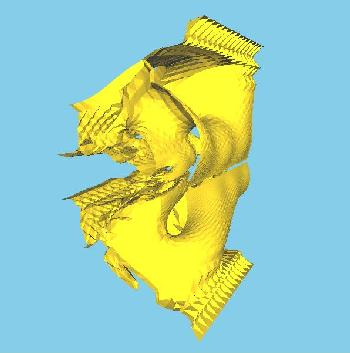# Datasets

## Tetrahedral Mesh Volume Data

#### (The images show some representative isosurfaces)

Format:
# vertices (n)
# cells (m)
vertex list of n vertices, each a tuple x y z f
--- (x,y,z) coordinates + scalar value f
cell list of m cells, each a tuple v1 v2 v3 v4
--- each vi is an index to the vertex list

## Time-Varying DataTpost10 and Tpost20 are of the same mesh, with 10 and 20 time steps respectively.

Format:
# vertices (n)
# time steps (t)
# cells (m)
vertex list of n vertices, each a tuple x y z f1 f2 ... ft
--- (x,y,z) coordinates + scalar values f1 ... ft
cell list of m cells, each a tuple v1 v2 v3 v4
--- each vi is an index to the vertex list

Tpost10
Tpost20